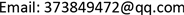1. 引言

2. 数据来源与研究方法2.1. 数据来源与数据处理

2.2. 研究方法

y i j = β o j + β 1 X i j + e i j (1)

β 0 j = γ 00 + μ 0 j (2)

y i j 和 X i j 分别为第j个学校中第i个学生应变量观测值和解释变量观测值。 γ 00 是 β 0 j 的平均值，为固定成份， μ 0 j 为水平2上( β 0 j )的随机成分，服从正态分布。 e i j 表示水平1上的随机误差，也服从正态分布。把(2)式代入(1)式并整理，得：

y i j = ( γ 00 + β 1 X i j ) + ( μ 0 j + e i j ) (3)

V a r ( y i j | γ 00 , β 1 , X i j ) = V a r ( μ 0 j + e 0 i j ) = V a r ( μ 0 j ) + V a r ( e 0 i j ) + C o v ( μ 0 j , e 0 i j ) = σ μ 0 2 + σ e 0 2

C o v ( μ 0 j + e i 1 j , μ 0 j + e i 2 j ) = C o v ( μ 0 j , μ 0 j ) = σ μ 0 2

ρ = σ μ 0 2 σ μ 0 2 + σ e 0 2 (4)

ICC测量了学校间方差占总方差的比例，实际上它反映了学校内个体间相关，即水平1单位(学生)在水平2单位(学校)中的聚集性或相似性。由于模型不止一个残差项，就产生了非零的组内相关。若ICC为0，表明数据不具备层次结构，可忽略学校的存在，即转化为传统的单水平模型；反之，若存在非零的 σ μ 0 2 ，则不能忽略学校的存在。

3. 结果分析3.1. 模型一：无解释变量(空模型)

Model test results when no explanatory variables are assume

00 = −0.267943)

( σ e 0 2 = 1.148051 ， σ u 0 2 = 0.337798 )

3.2. 模型二：引入变量

Model test results after introducing gender variable

[female = 0]−0.0764400.0535121612.658−1.4280.053−0.1814000.028519
[female = 1]0a0.....
（ γ 00 = − 0.229136 ， β 1 j = − 0.07644 ）

3.3. 模型三：引入家庭受教育程度变量

Model test results after introducing gender and education variable

[female = 0]−0.0701100.0531611614.155−1.3190.018−0.1743820.034163
[female = 1]0a0.....
[PE = 0]0.4743900.085345613.3025.5590.0000.3067870.641993
[PE = 1]0a0.....
（ γ 00 = − 0.427438 ， β 1 j = − 0.07011 ， β 2 = 0.474390 ）

4. 结论